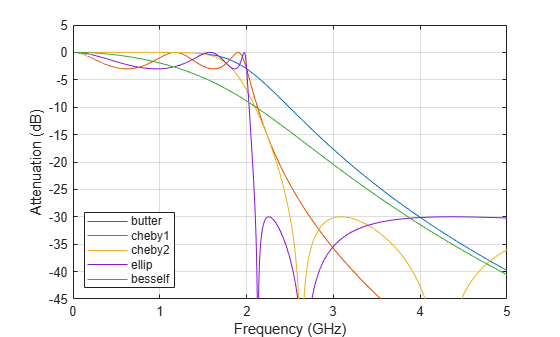Comparison of Analog IIR Lowpass Filters

Design a 5th-order analog Butterworth lowpass filter with a cutoff frequency of 2 GHz. Multiply by $2\pi$ to convert the frequency to radians per second. Compute the frequency response of the filter at 4096 points.

n = 5;
f = 2e9;

[zb,pb,kb] = butter(n,2*pi*f,'s');
[bb,ab] = zp2tf(zb,pb,kb);
[hb,wb] = freqs(bb,ab,4096);

Design a 5th-order Chebyshev Type I filter with the same edge frequency and 3 dB of passband ripple. Compute its frequency response.

[z1,p1,k1] = cheby1(n,3,2*pi*f,'s');
[b1,a1] = zp2tf(z1,p1,k1);
[h1,w1] = freqs(b1,a1,4096);

Design a 5th-order Chebyshev Type II filter with the same edge frequency and 30 dB of stopband attenuation. Compute its frequency response.

[z2,p2,k2] = cheby2(n,30,2*pi*f,'s');
[b2,a2] = zp2tf(z2,p2,k2);
[h2,w2] = freqs(b2,a2,4096);

Design a 5th-order elliptic filter with the same edge frequency, 3 dB of passband ripple, and 30 dB of stopband attenuation. Compute its frequency response.

[ze,pe,ke] = ellip(n,3,30,2*pi*f,'s');
[be,ae] = zp2tf(ze,pe,ke);
[he,we] = freqs(be,ae,4096);

Plot the attenuation in decibels. Express the frequency in gigahertz. Compare the filters.

plot(wb/(2e9*pi),mag2db(abs(hb)))
hold on
plot(w1/(2e9*pi),mag2db(abs(h1)))
plot(w2/(2e9*pi),mag2db(abs(h2)))
plot(we/(2e9*pi),mag2db(abs(he)))
axis([0 4 -40 5])
grid
xlabel('Frequency (GHz)')
ylabel('Attenuation (dB)')
legend('butter','cheby1','cheby2','ellip')The Butterworth and Chebyshev Type II filters have flat passbands and wide transition bands. The Chebyshev Type I and elliptic filters roll off faster but have passband ripple. The frequency input to the Chebyshev Type II design function sets the beginning of the stopband rather than the end of the passband.